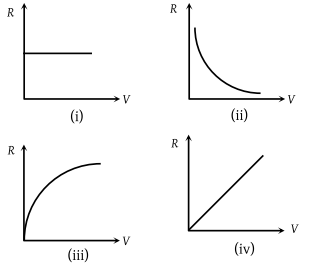The graph which represents the relation between the total resistance R of a multi range moving coil voltmeter and its full scale deflection V is(1) (i)

(2) (ii)

(3) (iii)

(4) (iv)

(4) For conversion of a galvanometer into a voltmeter

$\frac{V}{R+G}={i}_{g}$$\frac{V}{{R}_{V}}={i}_{g}$; where RV = R + G = Total resistance ⇒ ${R}_{V}=\frac{V}{{i}_{g}}$${R}_{V}\propto V$

Difficulty Level:

• 12%
• 35%
• 16%
• 39%